# How to Convert mmddyy to Date in Excel

This post will guide you how to convert non-standard date formats or text to a standard date in Excel. If you have a date with mmddyy format, and you want to change it as the normal date format as mm/dd/yyyy with Excel Formula. How do I convert the mmddyyyy text to normal date format in Excel 2013/2014.

Assuming that you have a list of data in range B1:B4 which contain date values as text format. and you can use one of the following methods to convert it as normal date format.

## Convert mmddyyyy Text to Date with Formula

To convert the mmddyyyy text to normal date format, you can use a formula based on the DATE function, the MID function and the RIGHT function. Just like this:

`=DATE(RIGHT(B1,4),LEFT(B1,2),MID(B1,3,2))`

Type this formula into a blank cell and press Enter key, and then drag the AutoFill Handle down to other cells to apply this formula to convert it.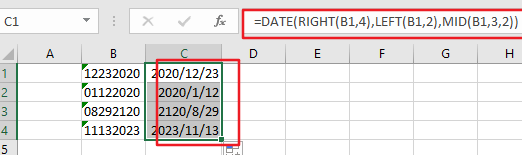## Convert mmddyy Text to Date with Text To Column Feature

You can also convert mmddyyyy Text to a normal date format with Text to Column feature in Excel. Just do the following steps:

Step1:select the range of cells that you want to convert, such as: B1:B4.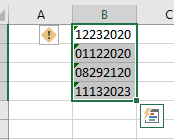Step2: go to Data tab in the Excel Ribbon, and click Text to Column command under Data Tools group. And the Convert Text to Columns dialog box will appear.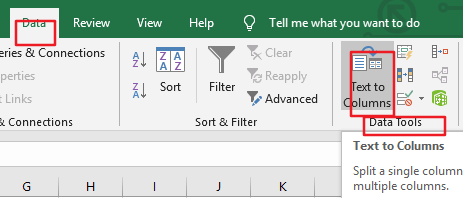Step3: select Delimited option under Original Data type section in the Convert Text to Columns dialog box, and click Next button. Click Next button again.

Step4: select Date option under the Column Data format, and then choose MDY from the drop down list box of Date. Then select on destination cell to place data. Click Finish button.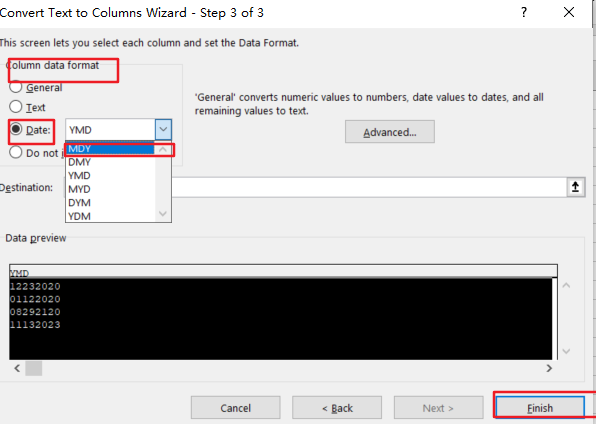Step5: you would see that the selected cells have been converted to a normal date format.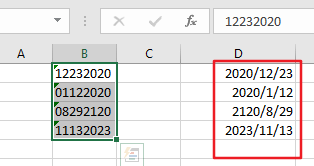## Convert mmddyy Text to Date with VBA Macro

If you want to convert mmddyyyy text to a normal date format quickly, you can also use an Excel VBA Macro to achieve the same result. Just do the following steps:

Step1: open your excel workbook and then click on “Visual Basic” command under DEVELOPER Tab, or just press “ALT+F11” shortcut.Step2: then the “Visual Basic Editor” window will appear.

Step3: click “Insert” ->”Module” to create a new module.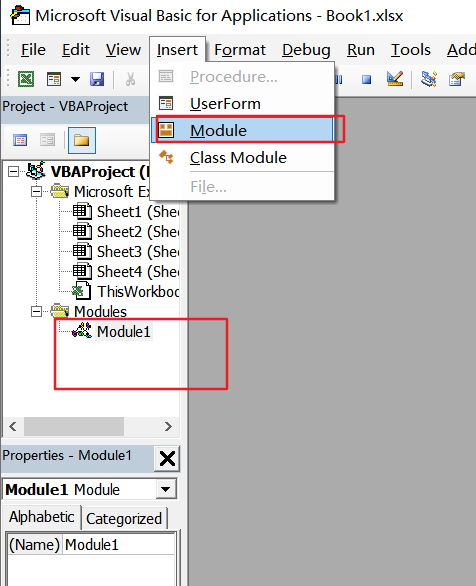el v

Step4: paste the below VBA code  into the code window. Then clicking “Save” button.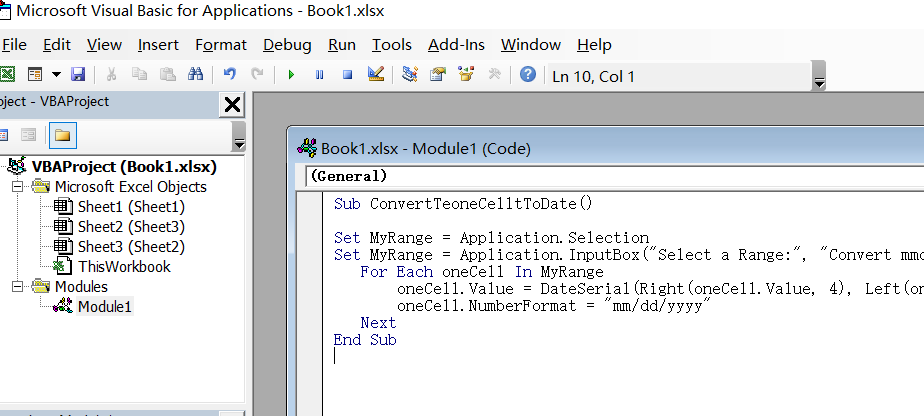```Sub ConvertTeoneCelltToDate()
Set MyRange = Application.Selection
Set MyRange = Application.InputBox("Select a Range:", "Convert mmddyyy to date", MyRange.Address, Type:=8)
For Each oneCell In MyRange
oneCell.Value = DateSerial(Right(oneCell.Value, 4), Left(oneCell.Value, 2), Mid(oneCell.Value, 3, 2))
oneCell.NumberFormat = "mm/dd/yyyy"
Next
End Sub```

Step5: back to the current worksheet, then run the above excel macro. Click Run button.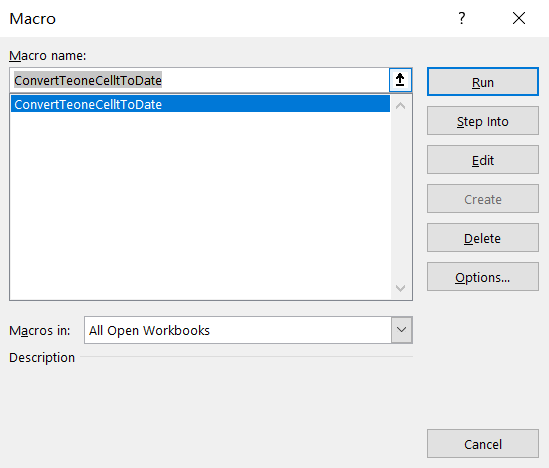Step6: select one range which contain text data that you want to convert, such as: B1:B4. Click OK button.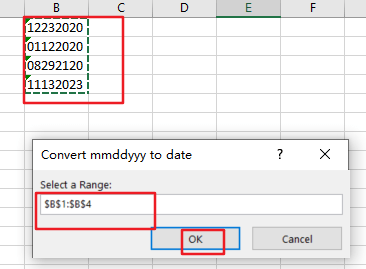Step7: Let’s see the result: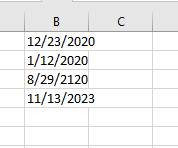### Related Functions

• Excel MID function
The Excel MID function returns a substring from a text string at the position that you specify.The syntax of the MID function is as below:= MID (text, start_num, num_chars)…
• Excel LEFT function
The Excel LEFT function returns a substring (a specified number of the characters) from a text string, starting from the leftmost character.The LEFT function is a build-in function in Microsoft Excel and it is categorized as a Text Function.The syntax of the LEFT function is as below:= LEFT(text,[num_chars])…
• Excel RIGHT function
The Excel RIGHT function returns a substring (a specified number of the characters) from a text string, starting from the rightmost character.The syntax of the RIGHT function is as below:= RIGHT (text,[num_chars])…
• Excel DATE function
The Excel DATE function returns the serial number for a date.The syntax of the DATE function is as below:= DATE (year, month, day)…

Related Posts

Find and Replace Multiple Values

This post will guide you how to find and replace multiple values at once with VBA macro or using formula  in Excel. How do I make multiple find and replace in Excel. Suppose that you have a few cells containing ...

VBA Macro For VLOOKUP From Another Sheet

In the previous post, you should know that how to fix or remove the #N/A error when using VLOOKUP formula to lookup value from another sheet. And this post will show you how to use VBA code to vlookup data ...

How To Insert Comments in Protected Worksheet in Excel

This post will show you how to allow comments in a protected worksheet in Excel. You can easily to insert comments into cells in a normal worksheet in Excel, but if want to insert a comment in a worksheet that ...

How To Convert Text to Upper Cases(Using VBA) in Excel

This post will show you how to switch from lower case to upper case in Excel. and I am going to show you two different ways of converting text to upper cases using formula or VBA macro in Excel 2013,Excel ...

How To Hide Every Other Row in Excel (Using VBA)

This post will show you how to hide alternate rows or columns in Excel or how to hide every third, fourth, fifth row or column in Excel. If you want to hide every other row in your current worksheet, how ...

How to Disable the Save As Prompt in Excel

This post will show you how to use a VBA Macro to save an Excel file and overwrite any existing file without a prompt so that you are going to get the little window that says file already exists do ...

How to Count Cells that Contain even or odd numbers in Excel

This post will guide you how to count the number of cells that contain odd or even numbers within a range of cells using a formula in Excel 2013/2016.How do I count cells that contain odd numbers through the use ...

How to Count Cells that Contain negative Numbers in Excel

This post will guide you how to count the number of cells that contain negative numbers within a range of cells using a formula in Excel 2013/2016.You can count the number of negative numbers in your data using easy functions ...

How to Count Cells Are Not Blank or Empty in Excel

This post will guide you how to count cells that are not blank or empty in a given range cells using a formula in Excel 2013/2016.How do I count the number of cells that are not blank in a particular ...

How to Count Cells Less Than a Specific Value in Excel

This post will guide you how to count the number of cells less than a particular numeric value in a given range cells using a formula in Excel 2013/2016. How do I count cells that are less than a specific ...

Sidebar